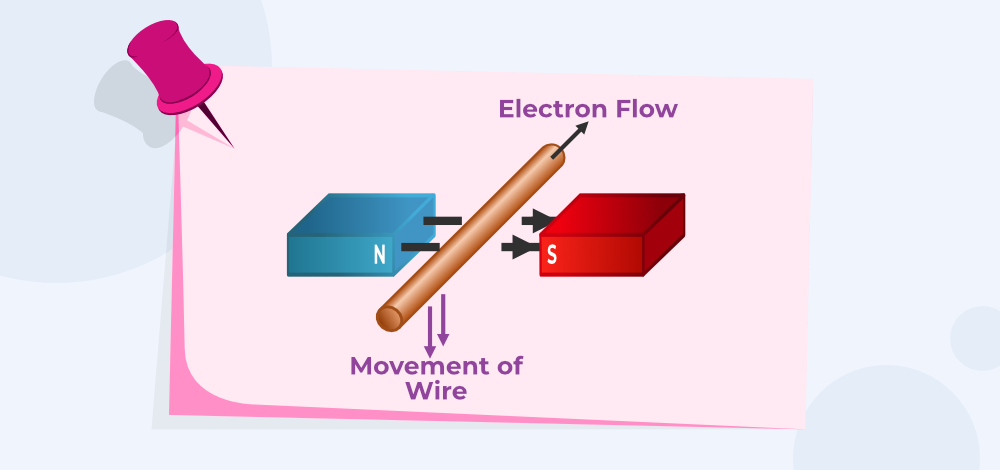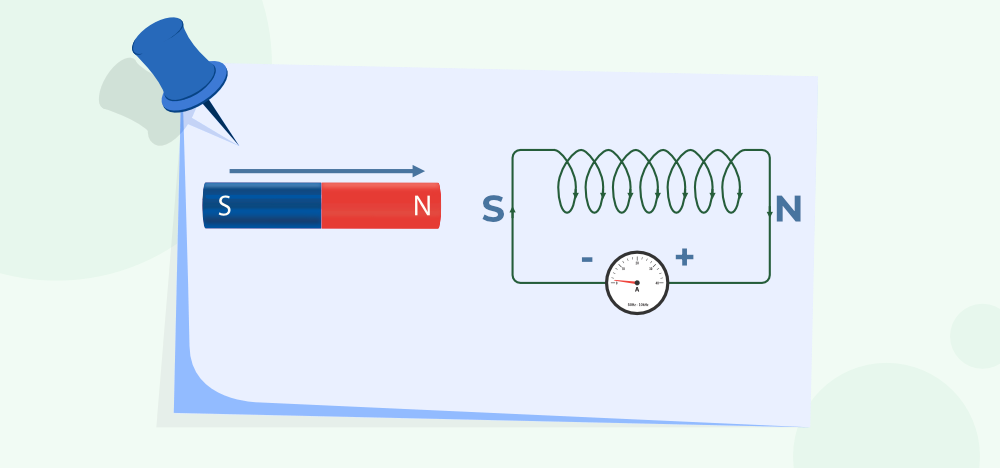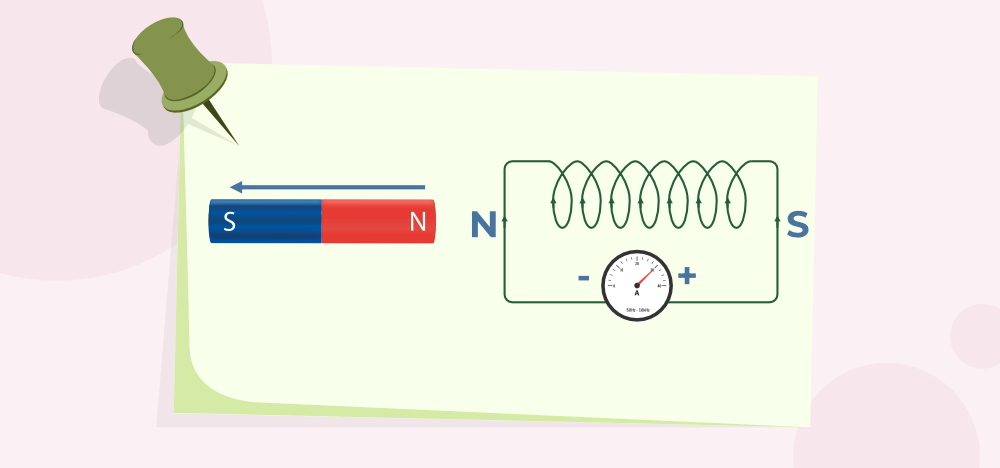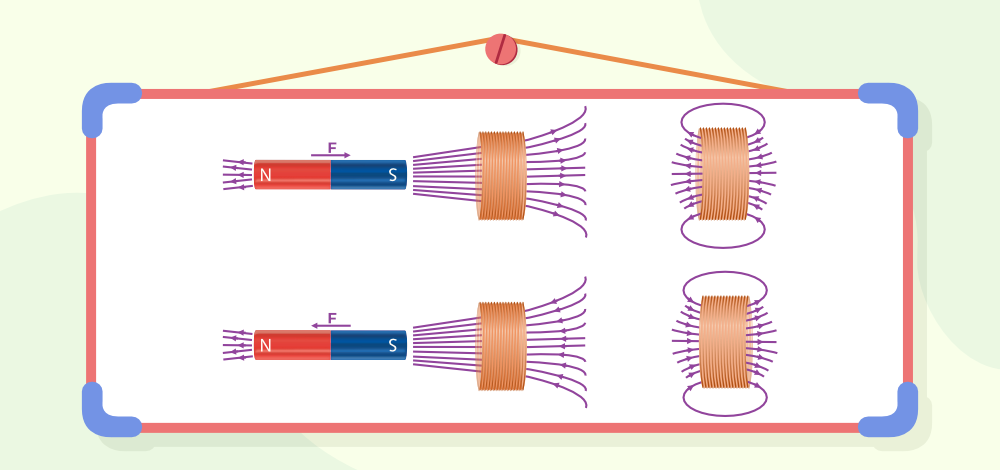GFG App
Open AppBrowser
Continue

Lenz law was given by the German scientist Emil Lenz in 1834 this law is based on the principle of conservation of energy and is in accordance with Newton’s third law. Lenz law is used to give the direction of induced current in the circuit.

## What is Lenz’s law?

The general definition of Lenz’s Law is,

“The induced current in a circuit due to Electromagnetic Induction always opposes the change in magnetic flux.”

It is a scientific law that specifies the direction of induced current but states nothing about its magnitude. The magnetic field associated with the closed circuit amplifies the induced current flow in such a way that it creates a magnetic field in the opposite direction of the original magnetic field. Thus, opposing the cause which produced it and stating its similarity with Newton’s third law.

## Lenz’s Law Formula

Lenz’s Law formula is stated from Faraday’s Law of Electromagnetic Induction. According to this law, EMF on the coil is calculated as,

E = -N(d∅/dt)

where,
negative sign indicates that the direction of induced emf is such that it opposes the change in magnetic flux)
E is the electromotive force
N is number of loops the coil made
d∅ is the change in magnetic flux
dt is change in time

## Lenz’s Law Experiment

Lenz’s law provides the direction of the induced electromotive force and current induced in the closed circuit. The experiments proved by Lenz to state its theory are,

The image given below shows a metallic conductor placed in a magnetic field.### First Experiment

First experiment by Lenz proved that the current flowing in the coil produces a magnetic field in the circuit and the strength of the magnetic field increases with an increase in the strength of the induced current. Also, this magnetic field produced opposes the original magnetic field i.e. the direction of the induced current is opposite to the original magnetic field.

### Second Experiment

Second experiment by Lenz states that the iron rod wound by the current-carrying wire and its left end behave as N-pole if moves towards the coil an induced current is produced in the coil.

### Third Experiment

Third experiment by Lenz states that if the coil is pulled towards the magnetic flux, the magnetic flux linked with the coil decreases as the area of the coil inside the magnetic field decreases. Now the induced current in the same direction opposes the motion of the coil according to Lenz’s law.

From the above experiments,  we can conclude that the current is produced when the magnet exerts the force in the loop and to resist the change, the current exerts a force on the magnet.

### What is Electromagnetic Induction?

It is the phenomenon of production of induced emf due to a change of magnetic flux (number of magnetic field lines) connected to a closed circuit called electromagnetic induction.

## Lenz’s Law Explanation

Lenz’s law is easily explained by two cases.

Case 1:As shown in the figure, when the North pole bar magnet is moved towards the coil, the induced current in the coil flows in the anticlockwise direction, when we see it from the magnet side. The face of the coil develops north polarity. As we know, that same pole repels, so the north pole-north pole repels. So, it opposes the motion of the North pole of a magnet.

Conclusion: The motion of the magnet increases the flux through the coil and flux will be generated in the opposite direction by the induced current.

Case 2:As shown in the figure when the North pole of a bar magnet is taken away from the coil, the induced current in the coil flows in the clockwise direction. The face of the coil develops South polarity. We know that opposite poles attract. So, the north pole and south polarity attract each other.

Conclusion: The motion of the magnet decreases the flux through the coil. The flux is generated in the same direction by induced current, hence opposing and increasing the flux.

## Lenz’s Law Applications

Lenz’s Law finds its importance in various cases and some of the most common  uses of Lenz’s law are,

• The braking system in trains works on the principle of Lenz’s law
• AC generators work on the principle of Lenz’s law
• Eddy currents are balanced using Lenz’s Law
• Metal Detectors, Card readers, and many other electronic devices use the concept of Lenz’s law for their application.

## Lenz’s law and Law of Conservation of Energy

Lenz’s law is a consequence of the law of conservation of energy. The law of conservation of energy states that energy can neither be created nor be destroyed, but it can be changed from one form to another form. Lenz’s law states that the direction of current is such that it opposes the change in the magnetic flux. So, extra effort is required to do work against opposing forces. This extra work leads to periodic changes in magnetic flux hence more current is induced. Thus, the extra effort gets converted into electrical energy only, which is nothing but the law of conservation of energy.

The magnetic flux increases as the North Pole of the magnet approaches it and drops as it is driven away in the activity above. In the first scenario, opposing the cause involves moving the magnet, and the face facing the coil gains North Polarity. The magnet’s north pole and the coil’s north pole repel each other. To counteract the force of repulsion, mechanical action must be done to bring the magnet towards the coil. This mechanical energy is transformed into electrical energy. Due to Joule’s Effect, this electrical energy is turned into heat energy.

The image given below shows the magnetic flux linked with the coil when a magnet is taken close or away from the coil.When the magnet is moved away from the coil, the coil’s nearer face obtains south polarity. In this instance, the produced emf will oppose the magnet’s outward motion. To resist the force of attraction between the North Pole of the magnet and the South Pole of the coil, mechanical labour must be done once more. This labour is transformed into electrical energy.

There is no mechanical work done if the magnet is not moved, hence no emf is induced in the coil.

As a result, Lenz’s Law is consistent with the law of conservation of energy.

Also, Check

## FAQs on Lenz’s Law

### Q1: What is Lenz’s law?

Lenz’s law states that the Induced current in a coil is in that direction which opposes the change in magnetic flux through the coil.

### Q2: Where is Lenz’s law used?

Lenz’s law is used to find the direction of induced current in any circuit. It works in accordance with Newton’s third law.

### Q3: What is the difference between Lenz’s law and Faraday’s law?

The difference between Faraday’s law and Lenz’s law can be explained through,

Lenz’s law states the direction of an induced current.

Faraday’s law states that the magnitude of the emf induced in a circuit is proportional to the rate of change of magnetic flux.

### Q4: What is the History of Lenz law?

A Baltic German physicist Heinrich Lenz proposed the Lenz Law in early 1900s

### Q5: Which law gives the direction of current in an AC generator?

Lenz’s law is used to provide the direction of current in an AC generator.

### Q6: How is Lenz’s law related to the law of conservation of energy?

Lenz’s law states that the induced EMF in the coil always opposes the cause which produces it which is in accordance with the law of conservation of energy.